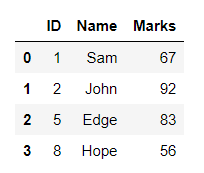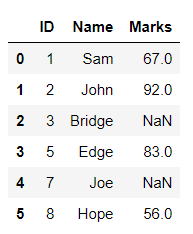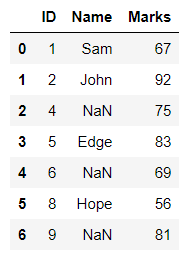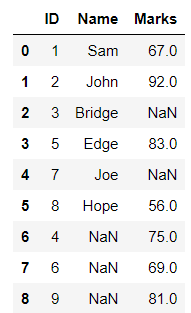# Merge two Pandas dataframes by matched ID number

• Last Updated : 05 Apr, 2021

In this article, we will see how two data frames can be merged based on matched ID numbers.

Approach

• Create a first data frame
• Create a second data frame
• Select Column to be matched
• Merge using the merge function

Syntax : DataFrame.merge(parameters)

• Display result

Given below are implementations to produce a required result with the use of the required parameter with an appropriate value.

Example:

## Python3

 `# import pandas as pd``import` `pandas as pd`` ` `# creating dataframes as df1 and df2``df1 ``=` `pd.DataFrame({``'ID'``: [``1``, ``2``, ``3``, ``5``, ``7``, ``8``], ``                    ``'Name'``: [``'Sam'``, ``'John'``, ``'Bridge'``,``                             ``'Edge'``, ``'Joe'``, ``'Hope'``]})`` ` `df2 ``=` `pd.DataFrame({``'ID'``: [``1``, ``2``, ``4``, ``5``, ``6``, ``8``, ``9``],``                    ``'Marks'``: [``67``, ``92``, ``75``, ``83``, ``69``, ``56``, ``81``]})`` ` `# merging df1 and df2 by ID``# i.e. the rows with common ID's get``# merged i.e. {1,2,5,8}``df ``=` `pd.merge(df1, df2, on``=``"ID"``)``print``(df)`

Output :Merged Dataframe

Merging two Dataframes with the ID column, with all the ID’s of the left Dataframe i.e. first parameter of the merge function. The ID’s which are not present in df2 gets a NaN value for the columns of that row.

Example 2 :

## Python3

 `# import pandas as pd``import` `pandas as pd`` ` `# creating dataframes as df1 and df2``df1 ``=` `pd.DataFrame({``'ID'``: [``1``, ``2``, ``3``, ``5``, ``7``, ``8``], ``                    ``'Name'``: [``'Sam'``, ``'John'``, ``'Bridge'``,``                             ``'Edge'``, ``'Joe'``, ``'Hope'``]})`` ` `df2 ``=` `pd.DataFrame({``'ID'``: [``1``, ``2``, ``4``, ``5``, ``6``, ``8``, ``9``],``                    ``'Marks'``: [``67``, ``92``, ``75``, ``83``, ``69``, ``56``, ``81``]})`` ` `# merging df1 and df2 by ID``# i.e. the rows with common ID's get merged``# with all the ID's of left dataframe i.e. df1``# and NaN for columns of df2 where ID do not match``df ``=` `pd.merge(df1, df2, on``=``"ID"``, how``=``"left"``)``print``(df)`

Output :Merged Dataframe

Merging two Dataframes with the ID column, with all the ID’s of the right Dataframe i.e. second parameter of the merge function. The ID’s which do not match from df1 gets a NaN value for that column.

Example 3 :

## Python3

 `# import pandas as pd``import` `pandas as pd`` ` `# creating dataframes as df1 and df2``df1 ``=` `pd.DataFrame({``'ID'``: [``1``, ``2``, ``3``, ``5``, ``7``, ``8``], ``                    ``'Name'``: [``'Sam'``, ``'John'``, ``'Bridge'``, ``                             ``'Edge'``, ``'Joe'``, ``'Hope'``]})`` ` `df2 ``=` `pd.DataFrame({``'ID'``: [``1``, ``2``, ``4``, ``5``, ``6``, ``8``, ``9``],``                    ``'Marks'``: [``67``, ``92``, ``75``, ``83``, ``69``, ``56``, ``81``]})`` ` `# merging df1 and df2 by ID``# i.e. the rows with common ID's get merged``# with all the ID's of right dataframe i.e. df2``# and NaN values for df1 columns where ID do not match``df ``=` `pd.merge(df1, df2, on``=``"ID"``, how``=``"right"``)``print``(df)`

Output :Merged Dataframe

Merging two Dataframes with the ID column, with all that match in both the dataframes.

Example 4 :

## Python3

 `# import pandas as pd``import` `pandas as pd`` ` `# creating dataframes as df1 and df2``df1 ``=` `pd.DataFrame({``'ID'``: [``1``, ``2``, ``3``, ``5``, ``7``, ``8``],``                    ``'Name'``: [``'Sam'``, ``'John'``, ``'Bridge'``,``                             ``'Edge'``, ``'Joe'``, ``'Hope'``]})`` ` `df2 ``=` `pd.DataFrame({``'ID'``: [``1``, ``2``, ``4``, ``5``, ``6``, ``8``, ``9``],``                    ``'Marks'``: [``67``, ``92``, ``75``, ``83``, ``69``, ``56``, ``81``]})`` ` `# merging df1 and df2 by ID``# i.e. the rows with common ID's get merged``# with all the ID's that match in both the Dataframe``df ``=` `pd.merge(df1, df2, on``=``"ID"``, how``=``"inner"``)``print``(df)`

Output :Merged Dataframe

Merging two Dataframes with the ID column, with all the ID’s of both the dataframes and NaN value for the columns where the ID is not found in both the dataframes.

Example 5 :

## Python3

 `# import pandas as pd``import` `pandas as pd`` ` `# creating dataframes as df1 and df2``df1 ``=` `pd.DataFrame({``'ID'``: [``1``, ``2``, ``3``, ``5``, ``7``, ``8``],``                    ``'Name'``: [``'Sam'``, ``'John'``, ``'Bridge'``,``                             ``'Edge'``, ``'Joe'``, ``'Hope'``]})`` ` `df2 ``=` `pd.DataFrame({``'ID'``: [``1``, ``2``, ``4``, ``5``, ``6``, ``8``, ``9``],``                    ``'Marks'``: [``67``, ``92``, ``75``, ``83``, ``69``, ``56``, ``81``]})`` ` `# merging df1 and df2 by ID``# i.e. the rows with common ID's get merged``# with all the ID's of both the dataframes``# and NaN values for the columns where the ID's ``# do not match``df ``=` `pd.merge(df1, df2, on``=``"ID"``, how``=``"outer"``)``print``(df)`

Output :Merged DataFrame

My Personal Notes arrow_drop_up